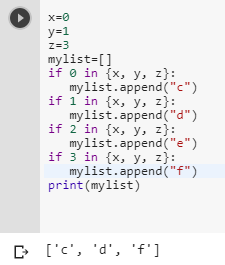1 view
in Python

I'm trying to make a function that will compare multiple variables to an integer and output a string of three letters. I was wondering if there was a way to translate this into Python. So say:

x = 0

y = 1

z = 3

mylist = []

if x or y or z == 0 :

mylist.append("c")

if x or y or z == 1 :

mylist.append("d")

if x or y or z == 2 :

mylist.append("e")

if x or y or z == 3 :

mylist.append("f")

which would return a list of

["c", "d", "f"]

Is something like this possible?

by (106k points)

The correct and fast way to test multiple variables against one value is by using set with separate if-statements so that Python will read each statement whether the former were True or False. Such as:

x=0

y=1

z=3

mylist=[]

if 0 in {x, y, z}:

mylist.append("c")

if 1 in {x, y, z}:

mylist.append("d")

if 2 in {x, y, z}:

mylist.append("e")

if 3 in {x, y, z}:

mylist.append("f")

print(mylist)This code will also work, but if you are comfortable using dictionaries you can clean this up by making an initial dictionary mapping the numbers to the letters you want, then just by using a for-loop:

x=0

y=1

z=3

mylist=[]

num_to_letters = {0: "c", 1: "d", 2: "e", 3: "f"}

for number in num_to_letters:

if number in {x, y, z}:

mylist.append(num_to_letters[number])

print(mylist)